Function Repository Resource:

# Game24Solutions

Find the list of solutions to the 24 Game by trying all possible options

Contributed by: Rauan Kaldybayev
 ResourceFunction["Game24Solutions"][ cards ] solve the 24 Game with an arbitrary number of cards.

## Details and Options

ResourceFunction["Game24Solutions"] takes the following options:
 "Goal" 24 the target integer "AllowPower" False whether to allow Power as a possible operation "OutputForm" String determines the format of the output
The option "OutputForm" accepts String, Hold and Inactive as values. The result will be a list of solutions with the specified form. This can make the output more convenient for further operations. However, this makes it harder to read and less aesthetic. Moreover, multiplication and division by 1 gets omitted if "OutputForm" is set to Hold, and division and subtraction are presented through multiplication and addition if "OutputForm" is set to Inactive.
ResourceFunction["Game24Solutions"] returns an empty list if the puzzle is impossible.

## Examples

### Basic Examples (2)

Use the function to find solutions to the 24 Game with four cards:

 In:=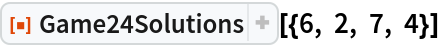Out=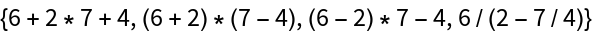Each element of the output list is a String expression representing a solution to the puzzle. If no solutions are found, an empty list is returned:

 In:=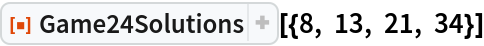Out=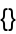Solve the puzzle with five cards given:

 In:=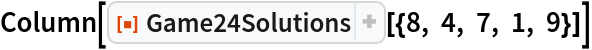Out=### Scope (2)

The values of all cards and the goal number must be positive integers:

 In:=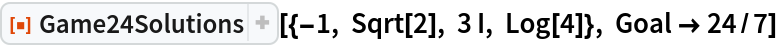Out=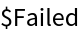Even though in the original 24 Game there is a limit to how high the value of a card can be, Game24Solve allows solutions with any positive integer values of cards:

 In:=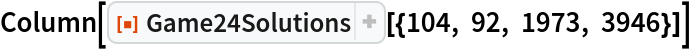Out=### Options (3)

OutputForm can be set to String, Hold or Inactive:

 In:=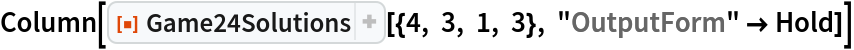Out=In:=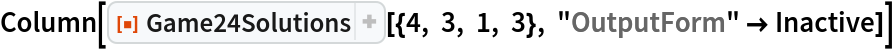Out=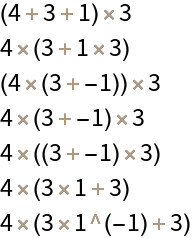Set the goal number to 36:

 In:=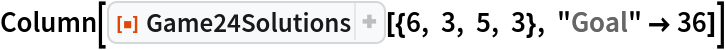Out=Solve the game when using powers is allowed. Note that allowing Power as an operation increases the computation time as there are more combinations to be checked:

 In:=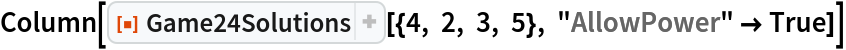Out=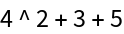## Publisher

Wolfram Summer Camp

## Version History

• 1.0.0 – 13 August 2020

## Author Notes

The running time of the function quickly rises with the number of input cards. Setting AllowPower True increases the computation time as well.